Engineering

# I have but one .condition(). Timeseries if-then-else with Timelion

Oh Timelion conditionals, we hardly know thee. Time to fix that. Introduced quietly into Timelion some time ago, the .condition() function is a powerhouse of, uh, power. Also, the title of this article is a total lie, I have more than one way I can call .condition(). The other is .if(). It works exactly the same as .condition(), but is much shorter, so from here on out we're using .if().

.if() allows us to change points based on a point-wise comparison. It supports less than (lt), less than or equal (lte), equal (eq), greater than (gt) and greater than or equal (gte) and compares points with either a number, or the same position in another series.

Note: For the purpose of this article I'm going to assume you've already used Timelion at some point. If you haven't, there's a handy-dandy tutorial built into the application itself. Click the friendly lion icon in Kibana 5 and he'll help you learn Timelion's simple syntax for timeseries taming. Alrighty, let's get down to business.

## Easy does it

Let's start simple. While .if() has four arguments, we are starting with these three for now

• operator One of lt, lte, eq, gt or gte. You might notice these are the same abbreviations that elasticsearch uses for the range query.
• if You'll be comparing each point in the original series to this value using the operator you specified.
• then Set the point to this value if the condition matches

In the following screenshots the green line will always be our original, and we'll use other colors for our modified series. Let's set every point less than 500 to 0

.es().if(lt, 500, null)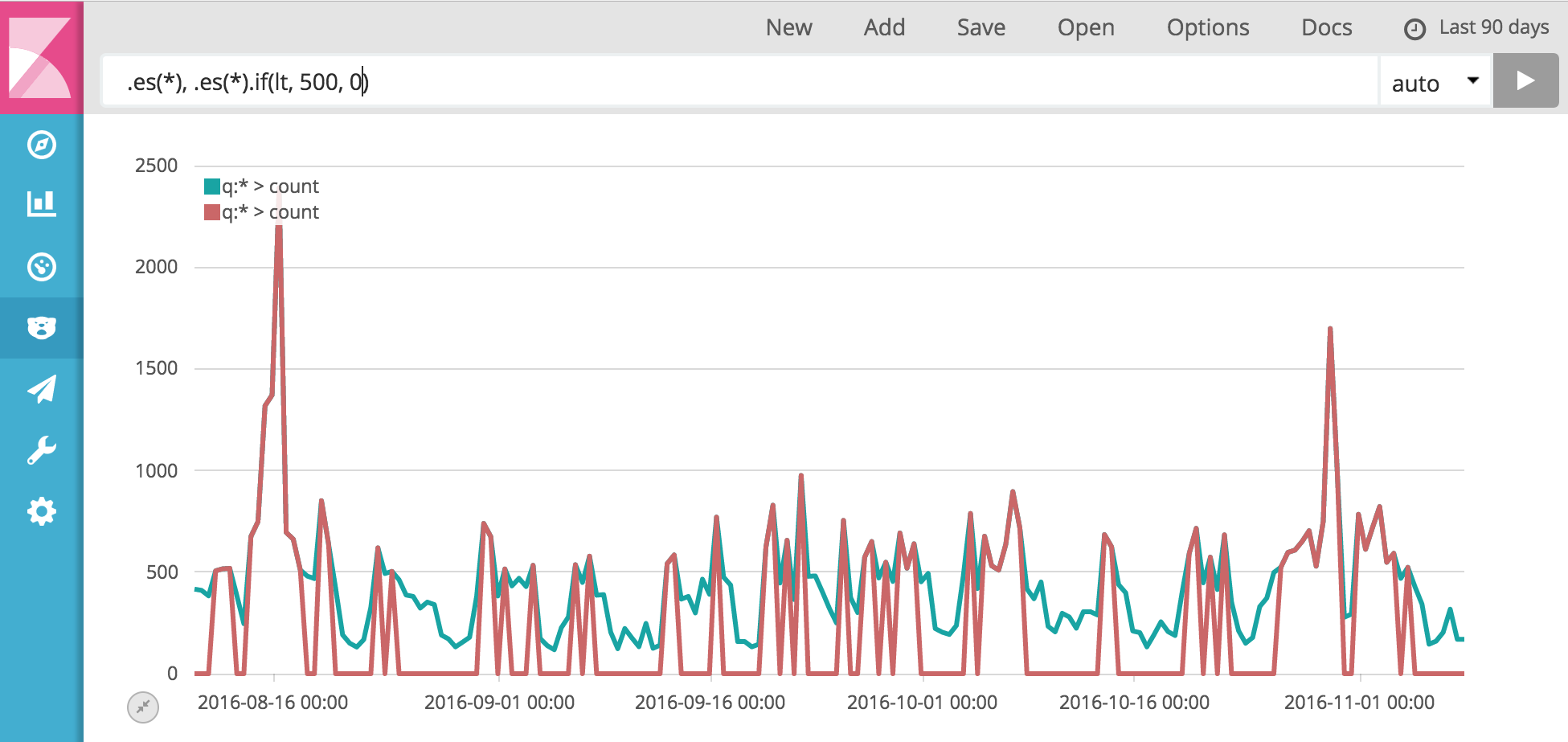Well that was simple. How about we set everything over, or equal to 500 to 1000, effectively making the series binary with 1000 representing points greater than or equal to 500, and 0 being points under 500. We could string together two .if() calls like this

.es().if(lt, 500, null).if(gte, 500, 1000)

But wait, we can make that shorter using the fourth argument to .if(). That fourth argument is else, and it sets the point to a value if the condition does not match. So try this instead

.es().if(lt, 500, 0, 1000)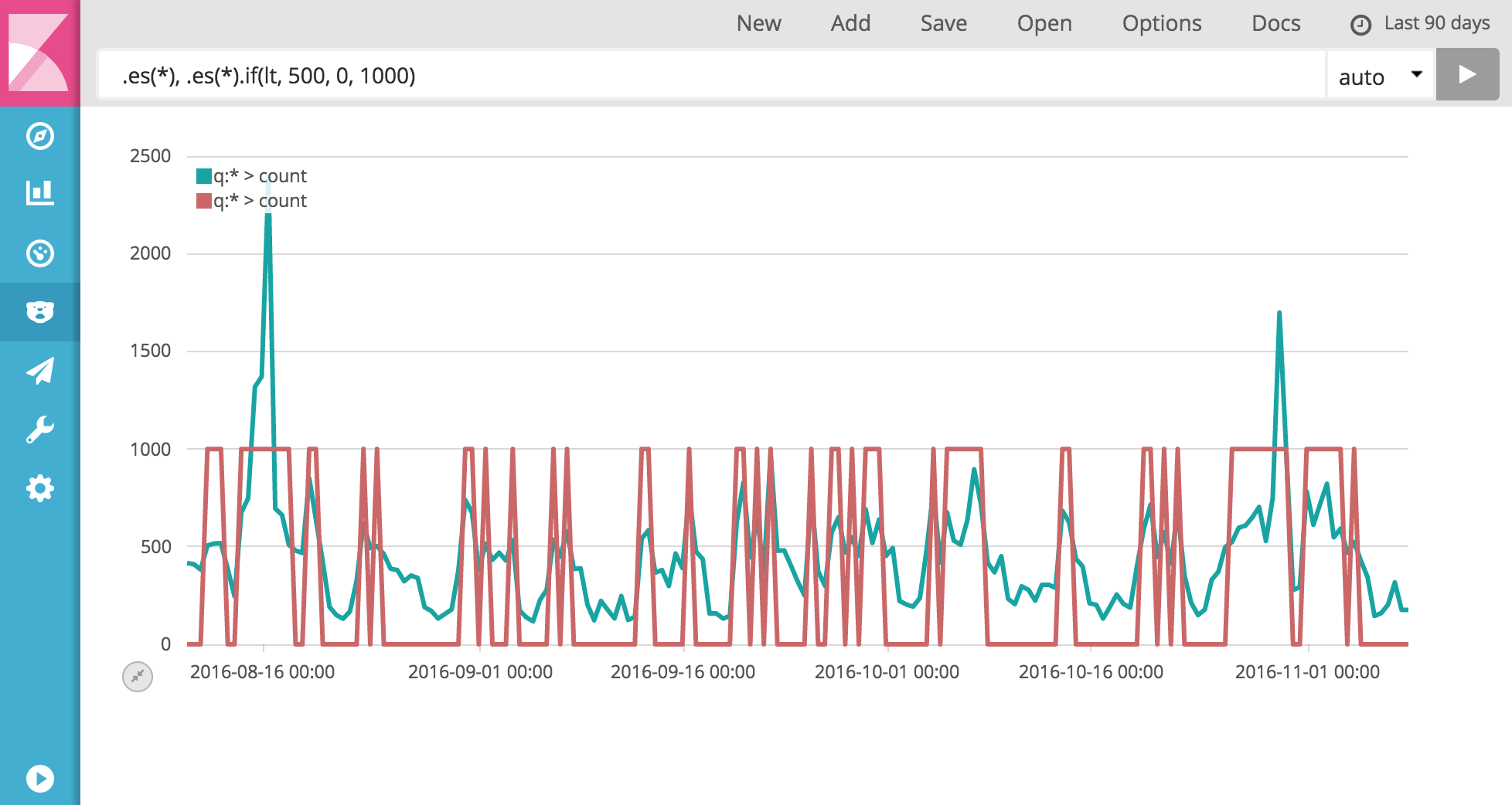Cool! Those are the basics, but .if() can munge more than static numbers. Read on brave soul.

## Dynamic? Dynamite!

So far we've compared points to a static number. What if we wanted to compare our series to another series. For example, draw a dot at 0 when the series exceeds its 10-point moving average, otherwise show nothing. Below you will see that we're passing .es().mvavg(10) as the if parameter. This will perform a point-wise comparison between the moving average and our original .es() series. The other part of our premise, showing nothing, can be accomplished by passing null to else.

.es().if(gt, .es().mvavg(10), 0, null).points()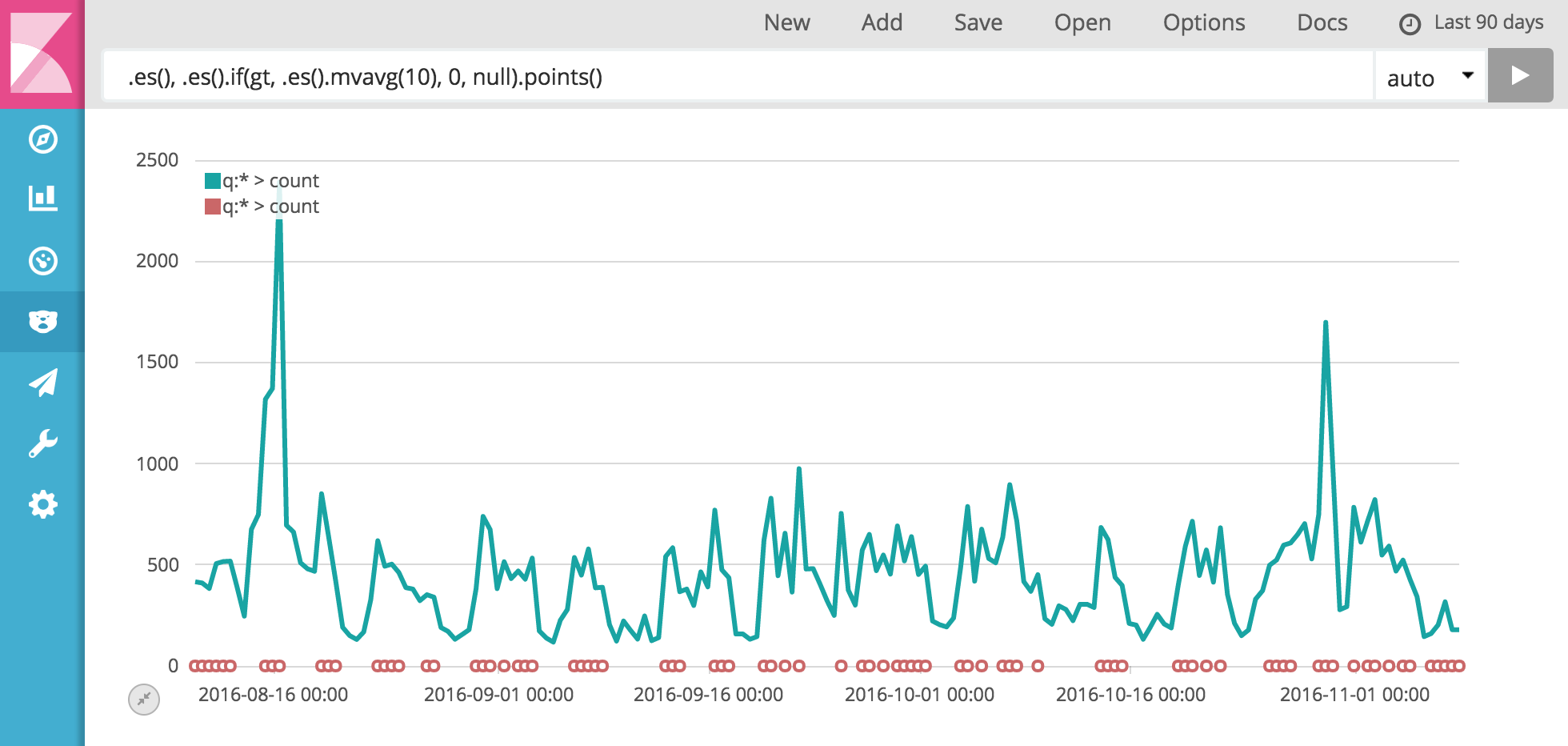Yeah, that's ok, but we can do better by also passing a series to then. We can pass back the original series and draw some bars pointing out the spots where the series exceeds the moving average by using .bars() instead of .points()

.es().if(gt, .es().mvavg(10), .es(), null).bars(3)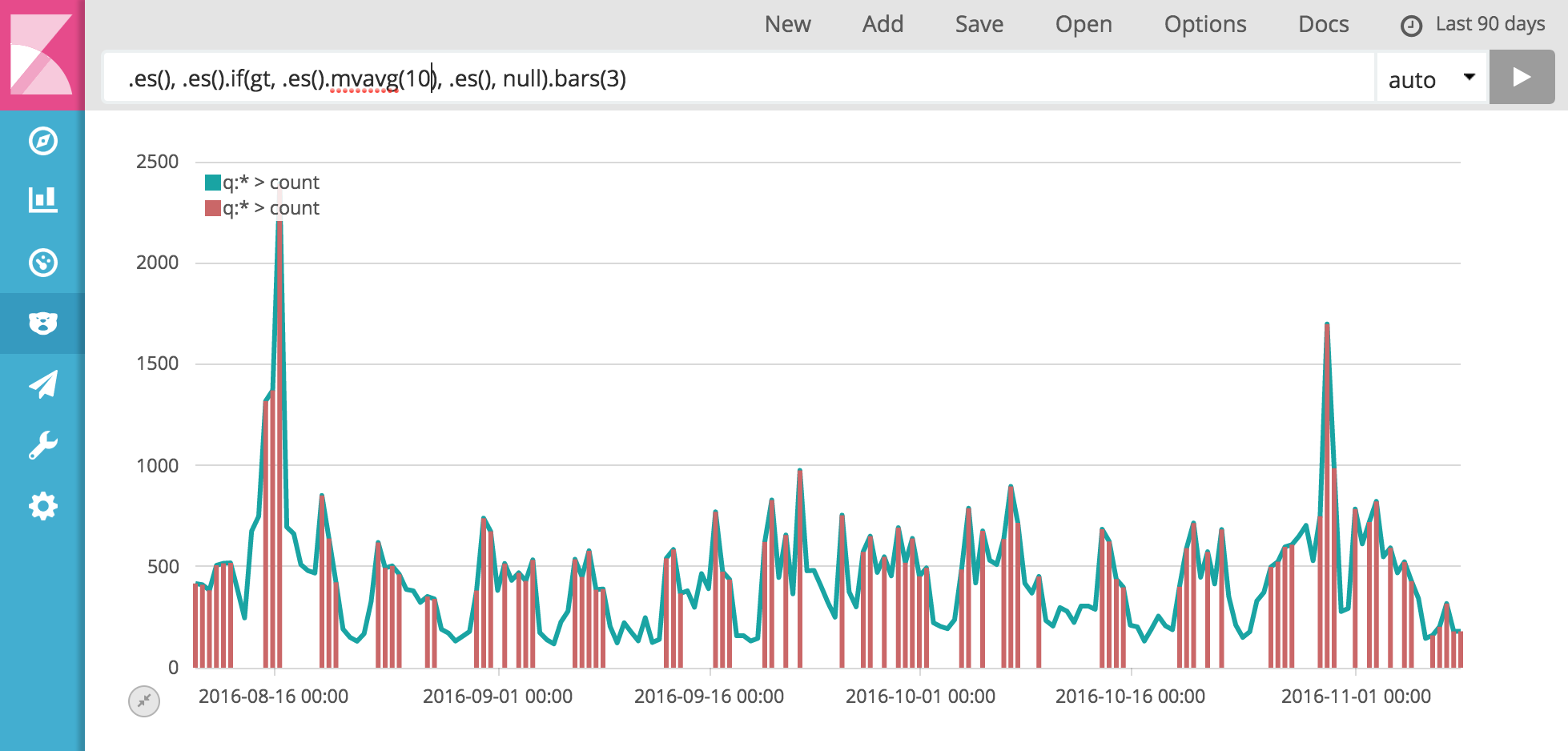## Altogether now

Now we take our new found knowledge and use it to get fancy, like drawing a chart that shades the area under the line green where we exceed the average, and red where we don't

.es().if(gt, .es().mvavg(10), .es(), null).bars(3).color(#BEDB39).label(over), .es().if(lte, .es().mvavg(10), .es(), null).bars(3).color(#c66).label(under)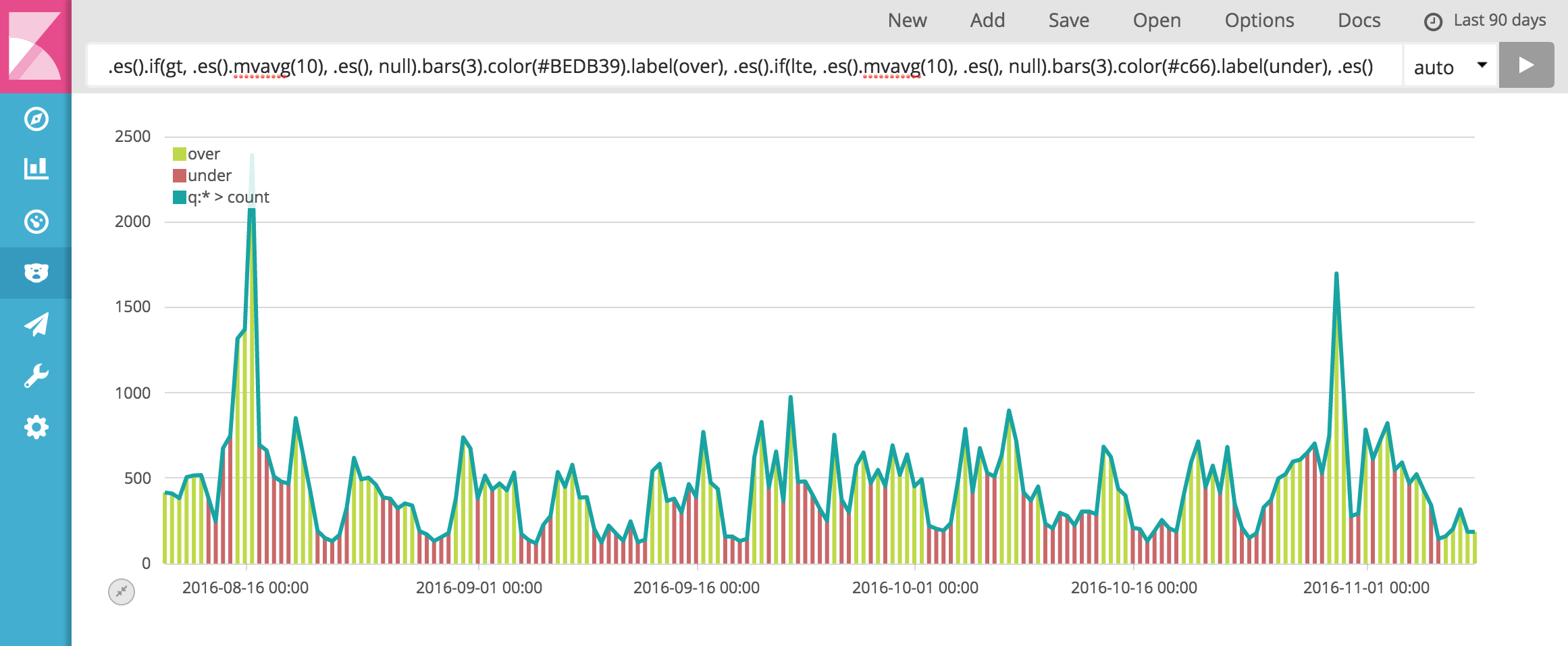Nice. Very nice. Still wondering what all this Timelion business is? Read the tutorial and still want more? Check out this video from the Elastic Paris meetup and I'll walk you through the nitty gritty. Plus you can watch me fumble with a microphone while trying to type with the other hand.